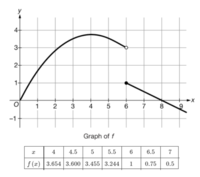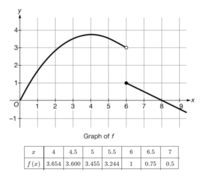# Need help with corrections.

#### Williian1

##### New member
Hi all,
So I understand for this question that f(6)=1 but I have troubles using that information with the f(6+h) part. Any clarification, guidance, examples, or just help in general would be greatly appreciated. Thanks!

For each of the following limits find the value or explain why it does not exist.

(i) lim ℎ→0+ $$\displaystyle 𝑓(6+ℎ)−𝑓(6)/h$$
(ii) lim ℎ→0 $$\displaystyle 𝑓(6+ℎ)−𝑓(6)/h$$
(iii) lim ℎ→0 $$\displaystyle 𝑓(6+ℎ)−𝑓(6)/h$$#### Subhotosh Khan

##### Super Moderator
Staff member
Hi all,
So I understand for this question that f(6)=1 but I have troubles using that information with the f(6+h) part. Any clarification, guidance, examples, or just help in general would be greatly appreciated. Thanks!

For each of the following limits find the value or explain why it does not exist.

(i) lim ℎ→0+ $$\displaystyle 𝑓(6+ℎ)−𝑓(6)/h$$
(ii) lim ℎ→0 $$\displaystyle 𝑓(6+ℎ)−𝑓(6)/h$$
(iii) lim ℎ→0 $$\displaystyle 𝑓(6+ℎ)−𝑓(6)/h$$

View attachment 24335
Please show us what you have tried and exactly where you are stuck.

Please follow the rules of posting in this forum, as enunciated at:#### Dr.Peterson

##### Elite Member
Hi all,
So I understand for this question that f(6)=1 but I have troubles using that information with the f(6+h) part. Any clarification, guidance, examples, or just help in general would be greatly appreciated. Thanks!

For each of the following limits find the value or explain why it does not exist.

(i) lim ℎ→0+ $$\displaystyle 𝑓(6+ℎ)−𝑓(6)/h$$
(ii) lim ℎ→0 $$\displaystyle 𝑓(6+ℎ)−𝑓(6)/h$$
(iii) lim ℎ→0 $$\displaystyle 𝑓(6+ℎ)−𝑓(6)/h$$

View attachment 24335
Presumably you meant

(i) lim ℎ→0+ [f(6+h)−f(6)]/h​
(ii) lim ℎ→0− [f(6+h)−f(6)]/h​
(iii) lim ℎ→0 [f(6+h)−f(6)]/h​

Do you see why that is important?

For (i), sketch the segment whose slope is represented by the difference quotient; what will this look like as h approaches zero? Then do the same for (ii), and make a conclusion for (iii).